# Improve your shiny application appearance

## Intro

In the previous post on Shiny ( here), we focused on the main functions to get started and build a complete application.

In this document, we’re going to focus only on the User Interface (UI), and look at more possibilities.

Recall that :

• We built the user interface in a `fluidPage()` function
• We used a type of layout to display a sidebar on the left and a main panel
• We used different types of inputs and outputs.

We’re going to :

• Understand the fluidPage layout to build more personalized layout
• Go through more inputs
• Customize the appearance with new themes

## Fluid Page Layout

A fluid page layout is composed of rows, each of them can be divided by columns.

To create rows, we use the function `fluidRow()` like this :

``````ui <- fluidPage(

titlePanel("My application"),

fluidRow(
"My first row"
),
fluidRow(
"My second row"
)

)

shinyApp(ui, server)``````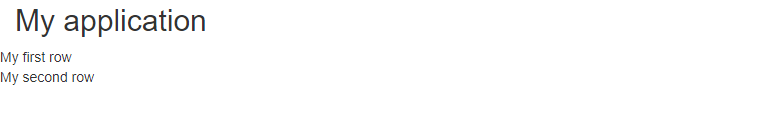Using fluidrow

To create a column, we use the function `column()` inside a `fluidRow()` (or a `fixedRow()`).

The width of a column can’t exceed 12, as the sum of all the column width. So for example, if we add a column of width 4, and a column of width 3, there will be an empty space of with 5 on the right.

The column function has also an offset parameter to shift the columns on the right.

Let’s look at an example :

``````ui <- fluidPage(
titlePanel("My application"),
fluidRow(
column(4,
textInput("text1", "Enter something")
),
column(3,
textInput("text2", "Enter something else")
)
),
fluidRow(
column(3,
textInput("text3", "Enter another thing"),
offset = 9
)
)
)

shinyApp(ui, server)``````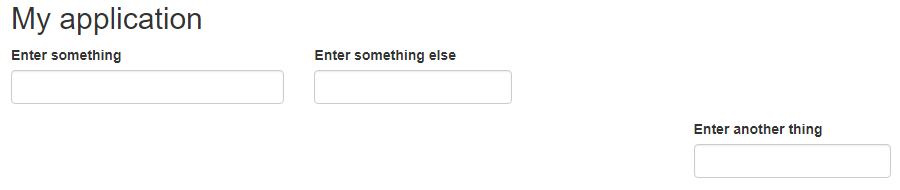fluidrow with columns for more advanced layout

To recap, a fluid page is a grid system, where the elements can be placed on the vertical axis by rows, and on the horizontal axis by column on a 12 unit wide range.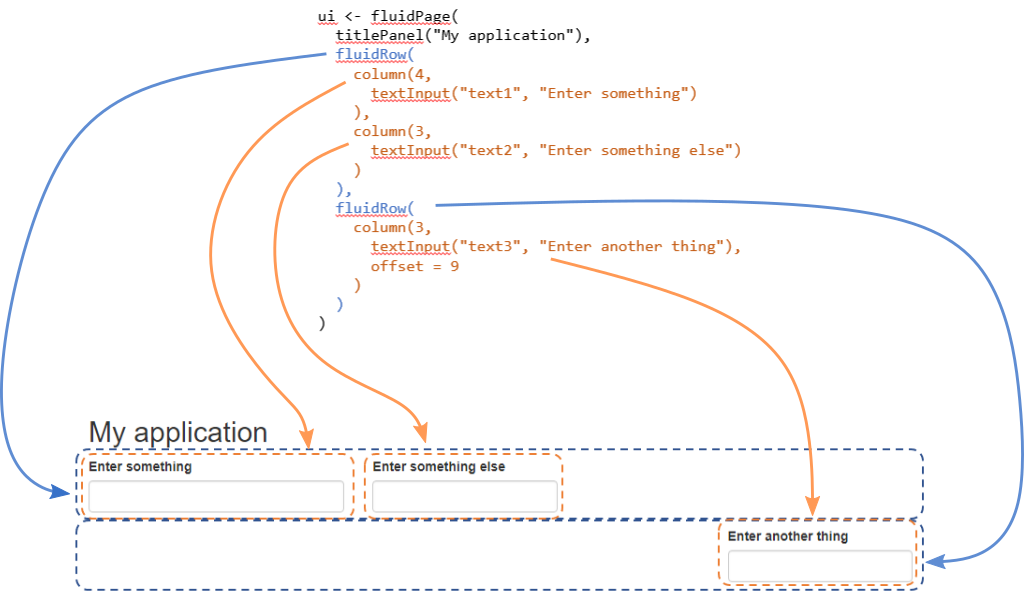understanding layouts in shiny

## Panels

Panels are used to group elements together, either because it looks better or because there is a functional logic.

### wellPanel

The wellPanel function group the elements included inside the panel and display them with a border and a background.

``````ui <- fluidPage(

titlePanel("My application"),

fluidRow(
column(5,
wellPanel(
textInput("text1", "Enter something"),
textInput("text2", "Enter something else")
) #wellPanel
),
column(3,
textInput("text3", "Enter another thing")
)
)

)

shinyApp(ui, server)``````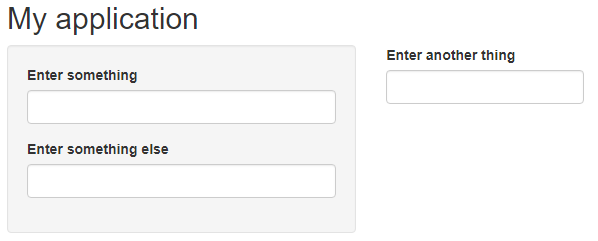grouping inputs with wellpanels

### tab Panel

We saw an example of using different tabs to display the result in the previous post.

This is easily done using `tabsetPanel()` function to wrap all the panels code, and `tabPanel()` for each tab.

`tabPanel()` has two parameters :

• the name of the tab to display
• the code of the output
``````library(palmerpenguins)

ui <- fluidPage(

sliderInput(inputId = "bill_length", label = "Select the range of bill length?", value = c(40,50), min = 32, max = 60),

tabsetPanel(
tabPanel(title = "Plot",
plotOutput("penguin_plot")
),
tabPanel(title = "Table",
tableOutput("penguin_table")
)
)

)

server <- function(input, output){

data <- reactive({
subset(penguins, bill_length_mm > input\$bill_length & bill_length_mm < input\$bill_length)
})

output\$penguin_plot <- renderPlot({
plot(data()\$bill_depth_mm, data()\$bill_length_mm, col = data()\$species)
})

output\$penguin_table <- renderTable({
data()
})
}

shinyApp(ui, server)``````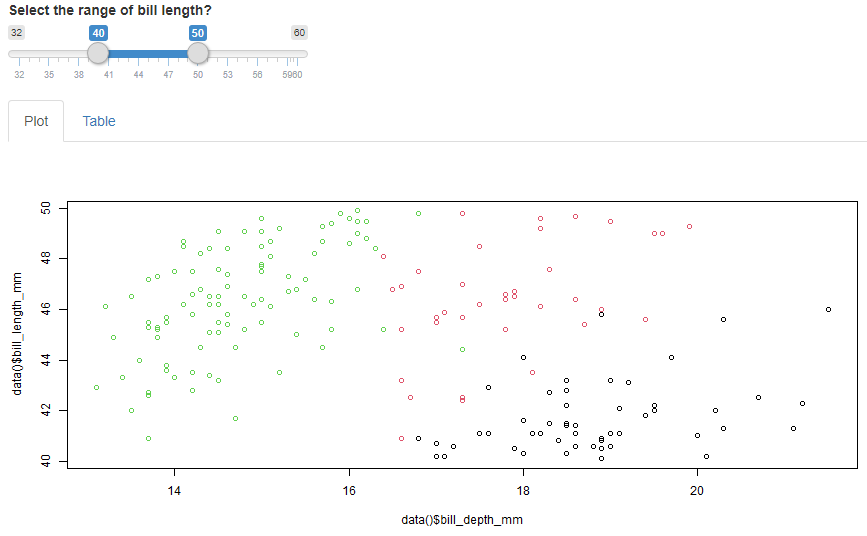using different tabs

## Fixed page vs fluid page

So far we’ve been using only `fluidPage()` (with `fluidRow()` ) to create the UI. There is the possibility of using `fixedPage()` instead. The difference between the two is that with fluidPage, the content adapts to a change of size without any limit, whereas the fixedPage has a limit size depending on the device.

The animated picture below illustrate this behavior :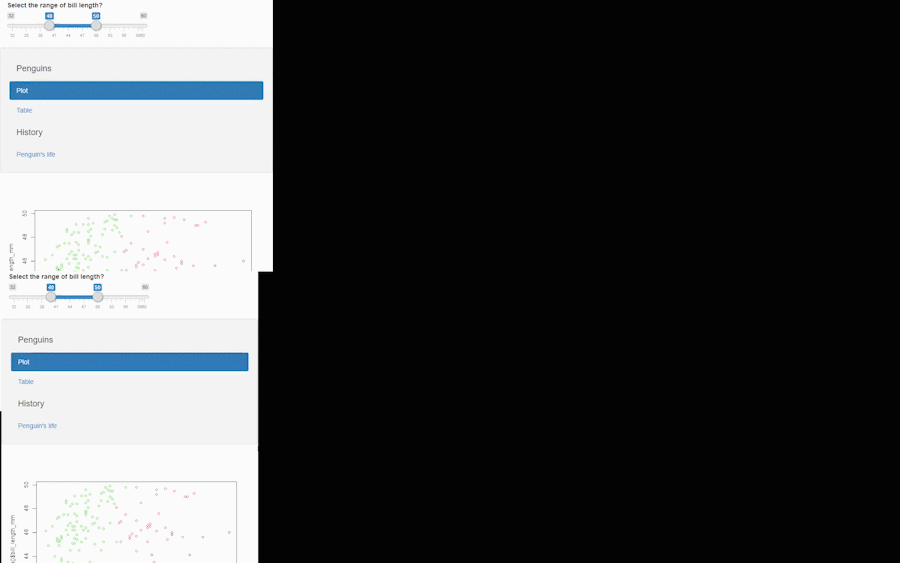difference between fixed page and fluid page

The case when you would want to use `fixedPage()` instead of `fluidPage()` is to make sure that the users will see the same layout even if their screen have different dimensions.

Note that you can’t use higher level layout such as `sidebarLayout()` while working with a fixed page.

While using `fixedPage()`, you need to use `fixedRow()` instead of `fluidRow()`

Example of a fixedPage UI :

``````ui <- fixedPage(

sliderInput(inputId = "bill_length", label = "Select the range of bill length?", value = c(40,50), min = 32, max = 60),

navlistPanel(
"Penguins",
tabPanel(title = "Plot",
plotOutput("penguin_plot")
),
tabPanel(title = "Table",
tableOutput("penguin_table")
),
"History",
tabPanel(title = "Penguin's life",
textOutput("penguin_text")
)
)

)``````##### Christophe Nicault
###### Information System Strategy Digital Transformation Data Science

I work on information system strategy, IT projects, and data science.